# How to return a vector in c++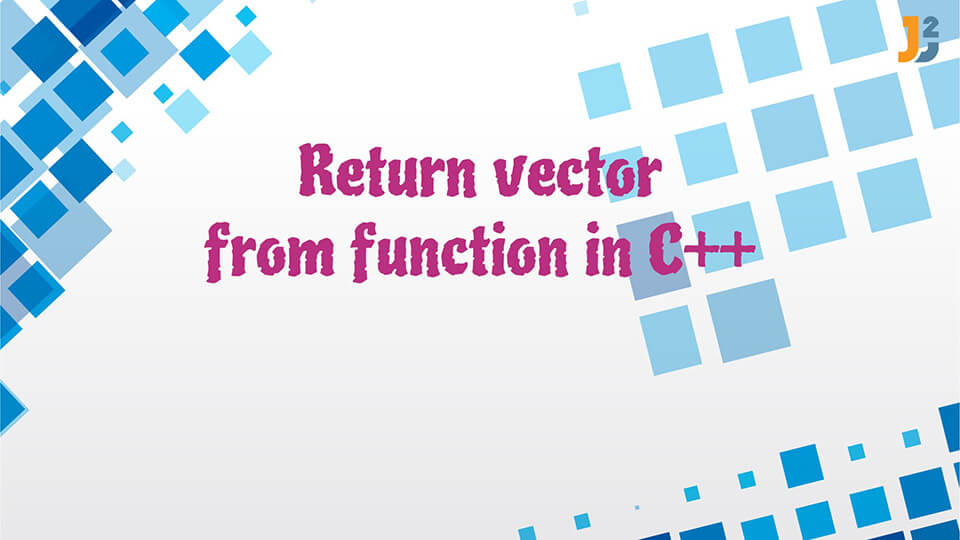In this post, we will see how to return a vector in C++.

vectors can be returned from a function in C++ using two methods: return by value and return by reference. In this article, we will discuss efficient ways to return a vector from a function in C++.

## Return by Value

It is a preferred method to return vector from function in C++. It can reduce the execution time and space because in this method, an object is not copied for returning a vector. It only points the pointer to the vector which will be returned.

Code:

Output:

Even elements are: 20 88 44 14

## Return by Reference

This method is better for returning large classes and structures. We will not return the reference of the local variable which is declared in the function because that can lead to dangling reference. In this method, we pass the vector by reference(& myFunction) and we will return as reference only.

Code:

Output:

Squares of the elements are: 400 2601 121 7744 1936 5929 196 9801

## Conclusion

In this article we discussed two efficient methods to return vector in C++. We can use return by value for smaller classes or structures. Return by reference method is only useful in the case of large structures and classes.

That’s all about how to return a vector in C++.
Happy Learning!!

## Related Posts

•19 May

### Get Type of Object in C++

Table of ContentsWhy Should We Determine the Type of Object in C++: Dynamic DispatchingGet Type of Object in C++Conclusion C++ is an object-oriented language where we often interact with objects of different types. The object interaction becomes more interesting when we add runtime polymorphism to it. The runtime polymorphism, also known as, dynamic dispatch of […]

•19 May

### Convert Vector to Array in C++

Table of ContentsVectors and Arrays in C++Convert Vector to Arrays in C++Using the for loop to convert vector to array in C++Using the copy() function to convert vector to array in C++Using the transform() function to convert vector to array in C++Using the data() function to convert vector to array in C++Using the & operator […]

•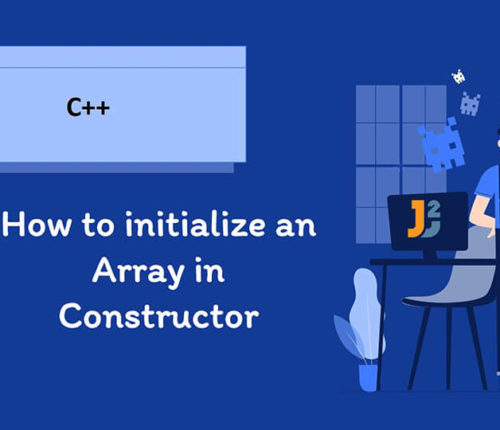23 April

### How to initialize an Array in Constructor in C++

Table of ContentsArraysConstructors of a ClassWays to initialize an array in Constructor in C++Initialize an array in Constructor With std::fill()Initialize an array in Constructor Using for LoopInitialize an array in Constructor Using Member Initializer ListConclusion This article discusses methods of initializing an array in constructor in C++. Let us first discuss arrays and constructors in […]

•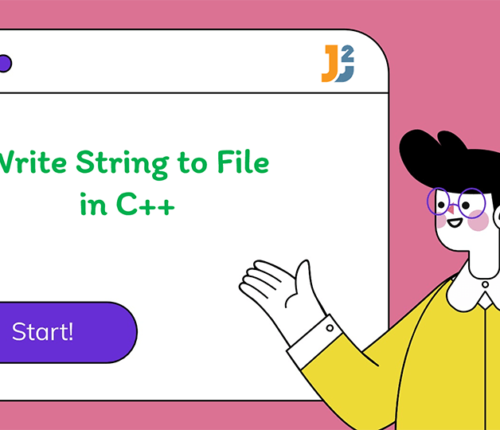31 March

### Write String to File in C++

Table of ContentsUsing the fstream Library to write String to File in C++Using the Insertion Operator (<<) With the fstream ObjectUsing the write() Function of the fstream LibraryUsing the fwrite() Function to write String to File in C++Conclusion The files are used to store the data permanently on a disk. This mitigates the problem of […]

•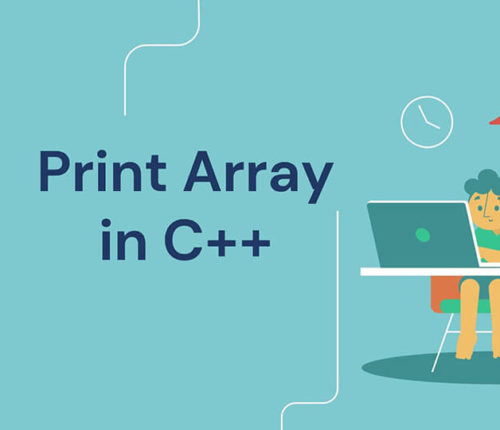23 March

### Print Array in C++

Table of ContentsIntroduction of ArraysDifferent Methods to Print an Array in C++print array in C++ by Traversing Indicesprint array in C++ Using for_each() Functionprint array in C++ Using Range-Based for Loopprint array in C++ Using Iteratorsprint array Using ostream_iterator() Function and copy() Functionprint array Using C++17 copy() Function and make_ostream_joiner() FunctionConclusion An array is a […]

•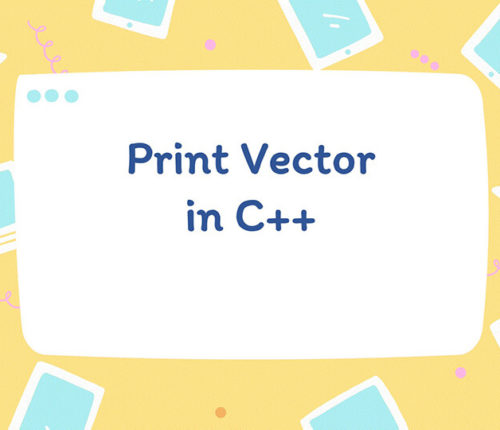23 March

### Print Vector in C++

Table of ContentsPrint Vector in C++ Using IndicesPrint Vector in C++ Using Range-Based for LoopPrint Vector in C++ Using for_each() FunctionPrint Vector in C++ Using IteratorPrint Vector in C++ by Overloading the Insertion Operator (<<)Print Vector in C++ Using copy() FunctionConclusion This article discusses the vector and how to print vector in C++. Vectors are […]

## Subscribe to our newletter

Get quality tutorials to your inbox. Subscribe now.